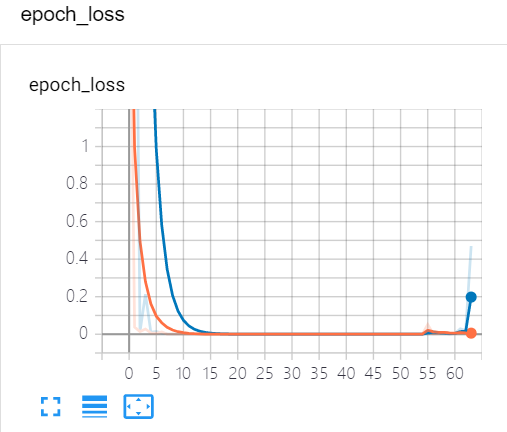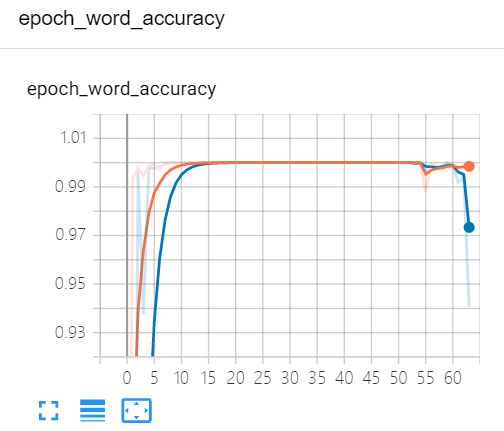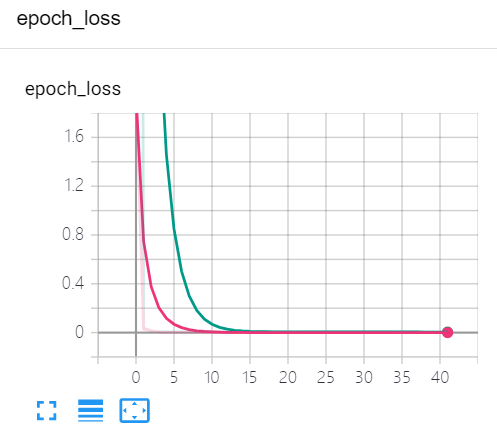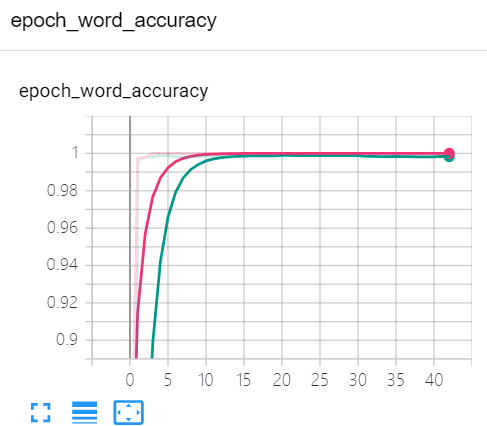# CRNN+CTC 识别验证码

GitHub: https://github.com/YouSmart2016/crnnvalidcode.git

## 第一步：生成数据集

``````def gen_captcha(img_dir,
num_per_image,
number,choices):
if os.path.exists(img_dir):
shutil.rmtree(img_dir,ignore_errors=True)
if not os.path.exists(img_dir):
os.makedirs(img_dir)
count=0
random_widths = list(range(64, 100, 1))
for _ in range(number):
for i in itertools.permutations(choices, num_per_image):
if count>=number:
break
else:
fn = os.path.join(img_dir, '%s_%s.jpg' % (captcha,count))
ima = ImageCaptcha(width=random_widths[int(random.random()*36)], height=32, font_sizes=(26, 28, 30))
ima.save(fn)
count += 1
``````

img_dir：保存图片的路径，num_per_image：每张图片生成几个字符，number：生成多少张，choices：表示字符集合

## 第二步：搭建网络模型

Model summary

Layer (type) Output Shape Param #
input_1 [(None, 32, 100, 1)] 0
conv2d (None, 32, 100, 64) 640
max_pooling2d (None, 16, 50, 64) 0
conv2d_1 (None, 16,50, 128) 73856
max_pooling2d_1 (None, 8, 25, 128) 0
conv2d_2 (None, 8, 25, 256) 294912
batch_normalization (None, 8, 25, 256) 1024
activation (None, 8, 25, 256) 0
conv2d_3 (None, 8, 25, 256) 590080
max_pooling2d_2 (None, 4, 25, 256) 0
conv2d_4 (None, 4, 25, 512) 1179648
batch_normalization_1 (None, 4, 25, 512) 2048
activation_1 (None, 4, 25, 512) 0
conv2d_5 (None, 4, 25, 512) 2359808
max_pooling2d_3 (None, 2, 25, 512) 0
conv2d_6 (None, 1, 24, 512) 1048576
batch_normalization_2 (None, 1, 24, 512) 2048
activation_2 (None, 1,24, 512) 0
reshape (None, 24, 512) 0
bidirectional (None,24, 512) 1574912
bidirectional_1 (None, 24, 512) 1574912
dense (None, 24, 11) 5643

Total params: 8,708,107

Trainable params: 8,705,547

Non-trainable params: 2,560

``````def vgg_style(input_tensor):

x = layers.Conv2D(64, 3, padding='same', activation='relu')(input_tensor)

x = layers.Conv2D(128, 3, padding='same', activation='relu')(x)

x = layers.Conv2D(256, 3, padding='same', use_bias=False)(x)
x = layers.BatchNormalization()(x)
x = layers.Activation('relu')(x)
x = layers.Conv2D(256, 3, padding='same', activation='relu')(x)
x = layers.MaxPool2D(pool_size=2, strides=(2, 1), padding='same')(x)

x = layers.Conv2D(512, 3, padding='same', use_bias=False)(x)
x = layers.BatchNormalization()(x)
x = layers.Activation('relu')(x)
x = layers.Conv2D(512, 3, padding='same', activation='relu')(x)

x = layers.MaxPool2D(pool_size=2, strides=(2, 1), padding='same')(x)

x = layers.Conv2D(512, 2, use_bias=False)(x)
x = layers.BatchNormalization()(x)
x = layers.Activation('relu')(x)
return x

def build_model(num_classes, image_width=None, channels=1):

img_input = keras.Input(shape=(32, image_width, channels))
x = vgg_style(img_input)
x = layers.Reshape((-1, 512))(x)

x = layers.Bidirectional(layers.LSTM(units=256, return_sequences=True))(x)
x = layers.Bidirectional(layers.LSTM(units=256, return_sequences=True))(x)
x = layers.Dense(units=num_classes)(x)
return keras.Model(inputs=img_input, outputs=x, name='CRNN')
``````

## 第三步：开始训练loss w/ 2 BiLSTMaccuracy w/ 2 BiLSTM

## 第四步：测试样本

4 位验证码测试图

Unbelievable，我没看错吧，居然全对，1 和 7 我都分不清，神经网络居然都能识别出来，太神奇了，简直了，再来 100 张测试一下。

4 位验证码测试图

1 位验证码测试图

2 位验证码测试图

3 位验证码测试图

5 位验证码测试图

6 位验证码测试图

4 位及 4 位以下验证码基本上 100% 识别，5 位验证码成功识别 94 张，错误 6 张，6 位验证码成功识别 71 张，错误 29 张，这样就结束了吗？远不止，请读者继续往下看。

## 第五步：模型优化

1 个 BiLSTM 代码

``````def vgg_style(input_tensor):
x = layers.Conv2D(64, 3, padding='same', activation='relu')(input_tensor)

x = layers.Conv2D(128, 3, padding='same', activation='relu')(x)

x = layers.Conv2D(256, 3, padding='same', use_bias=False)(x)
x = layers.BatchNormalization()(x)
x = layers.Activation('relu')(x)
x = layers.Conv2D(256, 3, padding='same', activation='relu')(x)
x = layers.MaxPool2D(pool_size=2, strides=(2, 1), padding='same')(x)

x = layers.Conv2D(512, 3, padding='same', use_bias=False)(x)
x = layers.BatchNormalization()(x)
x = layers.Activation('relu')(x)
x = layers.Conv2D(512, 3, padding='same', activation='relu')(x)

x = layers.MaxPool2D(pool_size=2, strides=(2, 1), padding='same')(x)

x = layers.Conv2D(512, 2, use_bias=False)(x)
x = layers.BatchNormalization()(x)
x = layers.Activation('relu')(x)
return x

def build_model(num_classes, image_width=None, channels=1):
img_input = keras.Input(shape=(32, image_width, channels))
x = vgg_style(img_input)
x = layers.Reshape((-1, 512))(x)
x = layers.Bidirectional(layers.LSTM(units=128, return_sequences=True))(x)
x = layers.Dense(units=num_classes)(x)
return keras.Model(inputs=img_input, outputs=x, name='CRNN')
``````loss w/ 1 BiLSTMaccuracy w/ 1 BiLSTM